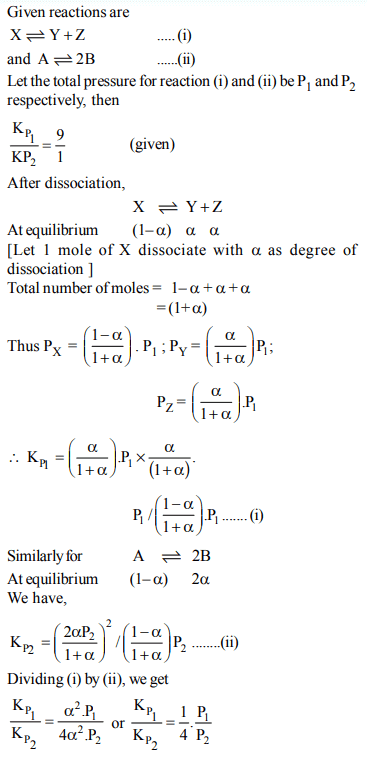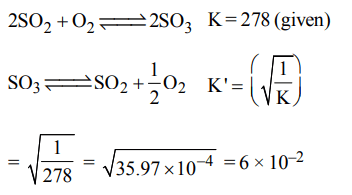## Chemical Equilibrium Questions and Answers Part-9

1. The values of $K_{P_{1}} and K_{P_{2}}$  for the reactions
$X\rightleftharpoons Y+Z$     ...(1)
and $A\rightleftharpoons 2B$     ...(2)
are in the ratio of 9 : 1. If degree of dissociation of X and A be equal, then total pressure at equilibrium (1) and (2) are in the ratio :
a) 3 : 1
b) 1 : 9
c) 36 : 1
d) 1 : 1

Explanation:2. The value of equilibrium constant of the reaction $HI\left(g\right)\rightleftharpoons\frac{1}{2}H_{2}\left(g\right)+\frac{1}{2}I_{2}$      is 8.0 The equilibrium constant of the reaction $H_{2}\left(g\right)+I_{2}\left(g\right)\rightleftharpoons2HI\left(g\right)$      will be:
a) $\frac{1}{16}$
b) $\frac{1}{64}$
c) 16
d) $\frac{1}{8}$

Explanation:3. In which of the following equilibrium $K_{c}$  and  $K_{p}$  are not equal?
a) $2 NO\left(g\right)\rightleftharpoons N_{2}\left(g\right)+O_{2}\left(g\right)$
b) $SO_{2}\left(g\right)+NO_{2}\left(g\right)\rightleftharpoons SO_{3}\left(g\right)+NO \left(g\right)$
c) $H_{2}\left(g\right)+I_{2}\left(g\right)\rightleftharpoons 2HI\left(g\right)$
d) $2C\left(s\right)+O_{2}\left(g\right)\rightleftharpoons 2CO_{2}\left(g\right)$

Explanation:4. The reaction $2A\left(g\right)+B\left(g\right)\rightleftharpoons 3C\left(g\right) +D\left(g\right)$       is began with the concentrations of A and B both at an initial value of 1.00 M. When equilibrium is reached, the concentration of D is measured and found to be 0.25 M. The value for the equilibrium constant for this reaction is given by the expression
a) $\left[\left(0.75\right)^{3}\left(0.25\right)\right]\div\left[\left(0.75\right)^{2}\left(0.25\right)\right]$
b) $\left[\left(0.75\right)^{3}\left(0.25\right)\right]\div\left[\left(1.00\right)^{2}\left(1.00\right)\right]$
c) $\left[\left(0.75\right)^{3}\left(0.25\right)\right]\div\left[\left(0.50\right)^{2}\left(0.75\right)\right]$
d) $\left[\left(0.75\right)^{3}\left(0.25\right)\right]\div\left[\left(0.50\right)^{2}\left(0.25\right)\right]$

Explanation:5. Given that the equilibrium constant for the reaction $2SO_{2}\left(g\right)+O_{2}\left(g\right)\rightleftharpoons2SO_{3}\left(g\right)$      has a value of 278 at a particular temperature. What is the value of the equilibrium constant for the following reaction at the same temperature ?
$SO_{3}\left(g\right)\rightleftharpoons SO_{2}\left(g\right)+\frac{1}{2}O_{2}\left(g\right)$
a) $1.8 × 10^{-3}$
b) $3.6 × 10^{-3}$
c) $6.0 × 10^{-2}$
d) $1.3 × 10^{-5}$

Explanation:6. Given the reaction between 2 gases represented by $A_{2} and B_{2}$  to give the compound $AB\left(g\right)$
$A_{2}\left(g\right) + B_{2}\left(g\right)\rightleftharpoons AB\left(g\right)$
At equilibrium, the concentration
of $A_{2}=3.0 × 10^{-3}M$
of $B_{2}=4.2 × 10^{-3}M$
of $AB_{2}=2.8 × 10^{-3}M$
If the reaction takes place in a sealed vessel at 527°C, then the value of $K_{C}$ will be :
a) 2.0
b) 1.9
c) 0.62
d) 4.5

Explanation:7. Change in volume of the system does not alter the number of moles in which of the following equilibria?
a) $N_{2}\left(g\right)+O_{2}\left(g\right)\rightleftharpoons2NO\left(g\right)$
b) $PCl_{5}\rightleftharpoons PCl_{3}\left(g\right)+CI_{2}\left(g\right)$
c) $N_{2}\left(g\right)+3H_{2}\left(g\right)\rightleftharpoons2NH_{3}\left(g\right)$
d) $SO_{2}CI_{2}\left(g\right)\rightleftharpoons SO_{2}\left(g\right)+CI_{2}\left(g\right)$

Explanation: In this reaction the ratio of number of moles of reactants to products is same i.e. 2 : 2, hence change in volume will not alter the number of moles.

8. In which of the following reactions, increase in the volume at constant temperature does not affect the number of moles at equilibrium.
a) $2NH_{3}\rightarrow N_{2}\left(g\right)+3H_{2}\left(g\right)$
b) $C\left(s\right)+\left(1/2\right)O_{2}\left(g\right)\rightarrow CO\left(g\right)$
c) $H_{2}\left(g\right)+O_{2}\left(g\right)\rightarrow H_{2}O_{2}\left(g\right)$
d) None of these

Explanation: In (a), (b) and (c) no. of mole of products is not equal to no. of mole of reactants

9. For the reaction
$CO\left(g\right)+\left(1/2\right)O_{2}\left(g\right)\rightleftharpoons CO_{2}\left(g\right),K_{p}/K_{c}$        is
a) RT
b) $\left(RT\right)^{-1}$
c) $\left(RT\right)^{-1/2}$
d) $\left(RT\right)^{1/2}$

Explanation:10. For the reaction equilibrium
$N_{2}O_{4}\left(g\right) \rightleftharpoons2NO_{2}\left(g\right)$
the concentrations of $N_{2}O_{4}and NO_{2}$   at equilibrium are $4.8 ×10^{-2} and 1.2 ×10^{-2}mol L^{-1}$  respectively. The value of Kc for the reaction is
a) $3 ×10^{-1}mol L^{-1}$
b) $3 ×10^{-3}mol L^{-1}$
c) $3 ×10^{3}mol L^{-1}$
d) $3 ×10^{2}mol L^{-1}$